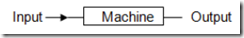## Tuesday, 4 July 2017

Dear Aspirant,

Input – Output  Preparation tips for Bank exams IBPS SBI

Introduction: The questions of Input-Output in the PO exams are very common and can be very much  scoring within few minutes. These questions are pattern based in which you have to find   the hidden pattern and then answer the given questions.

Here we will practice the questions of Input-Output
These are the problems that test your aptitude. You will be given some input and a machine (its   working) using some examples. You have to study this working and accordingly process the data  given to you.In the questions related to this topic, you are expected to be the “machine”. You would be told  what  processing  has  to  be  done.  You  would  be  given  the  input,  your  job  would  be  give  the  output in accordance to the input.
In the topic of input output there are primarily four types of operation that can be asked in a  particular question. The four operations are as follows:

1.  Ordering input according to given criteria, e.g. alphabetically, in increasing/decreasing order  etc.

2.  Mathematical  Manipulations  on  the  output  which  would  in  this  case  obviously  be  numerical. The operations could be doubling, squaring, adding.

3.  Shifting  or  Interchanging  positions  of  characters/alphabets/words  etc.,  in  the  input  according to questions e.g. ‘shift 1st character to last’ or ‘interchange 1st & last’ etc.
4.  Others like making an alphabet capital, replacing alphabet by corresponding number etc.